Finding General Solutions

Chapter 3 Class 11 Trigonometric Functions
Concept wise

For general solutions

We must learn

For sin x = sin y,

x = nπ + (–1) n y, where n ∈ Z

For cos x = cos y ,

x = 2nπ ± y, where n ∈ Z

For tan x = tan y,

x = nπ + y, where n ∈ Z

Note : Here n ∈ Z   means n is an integer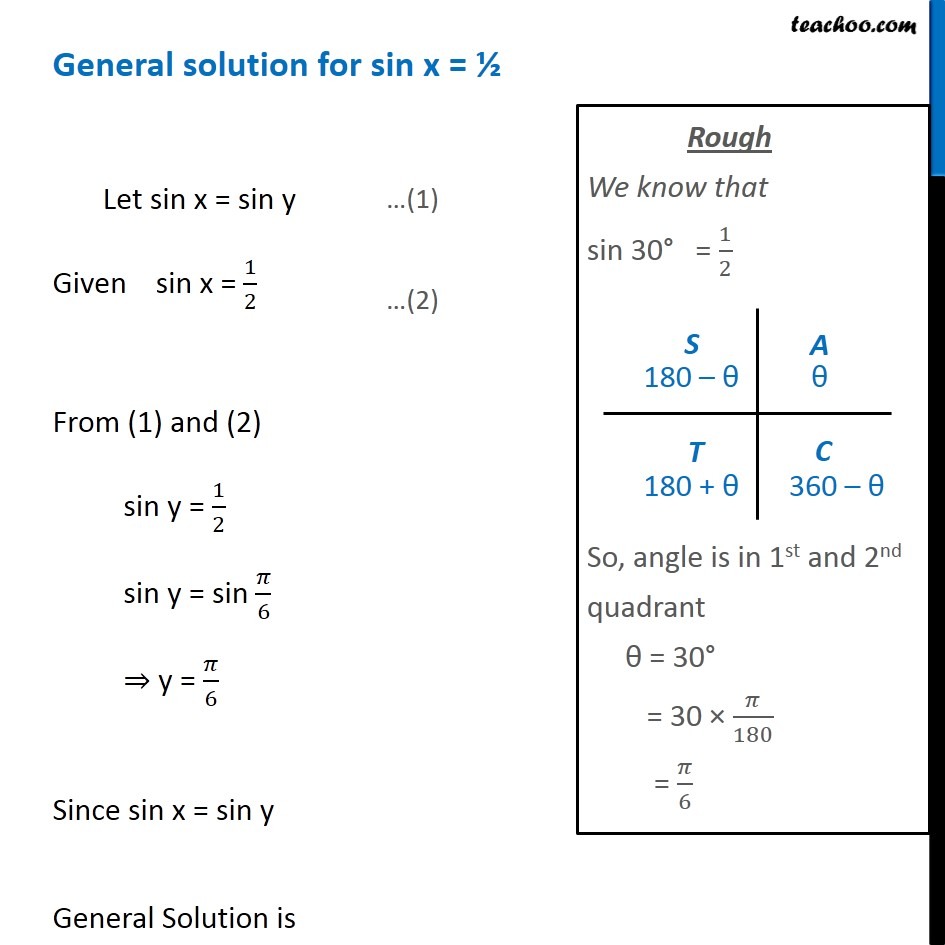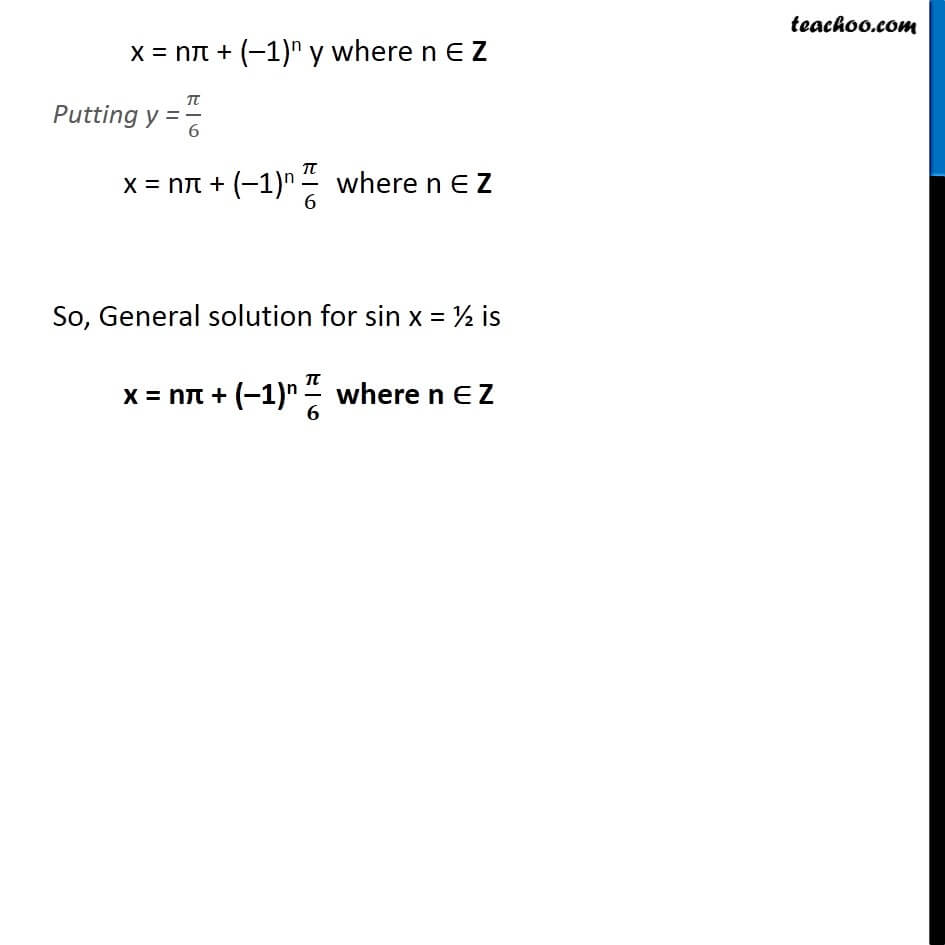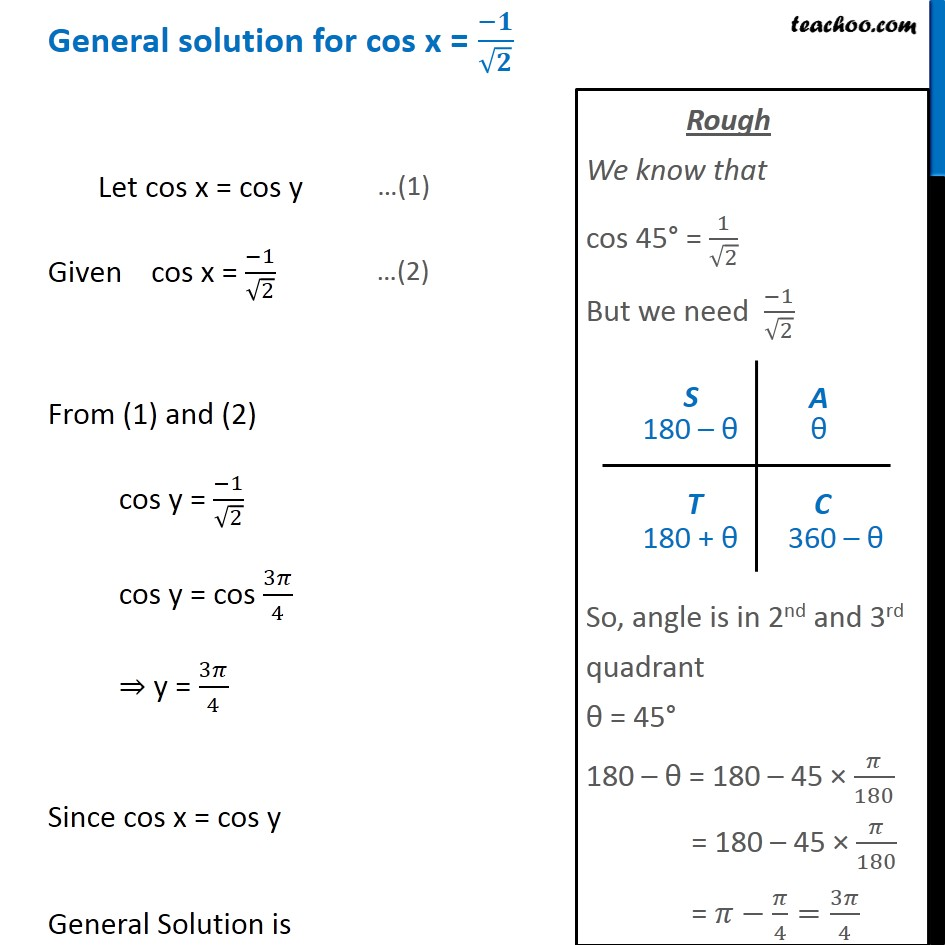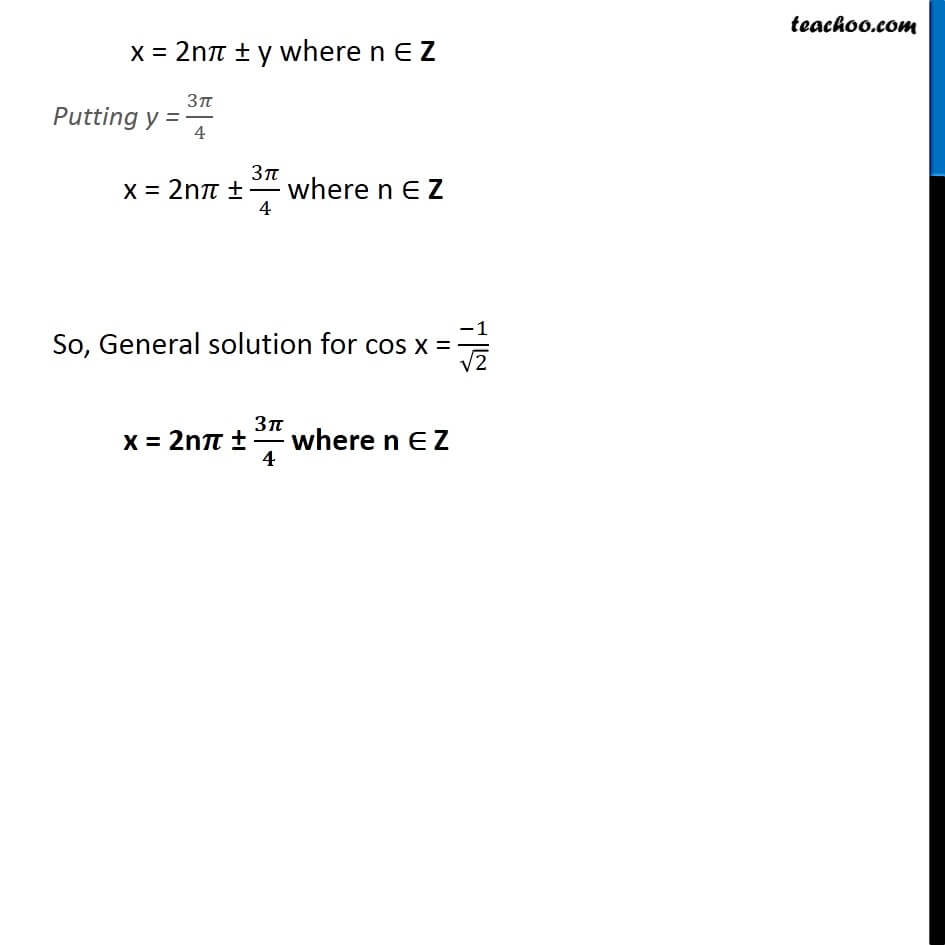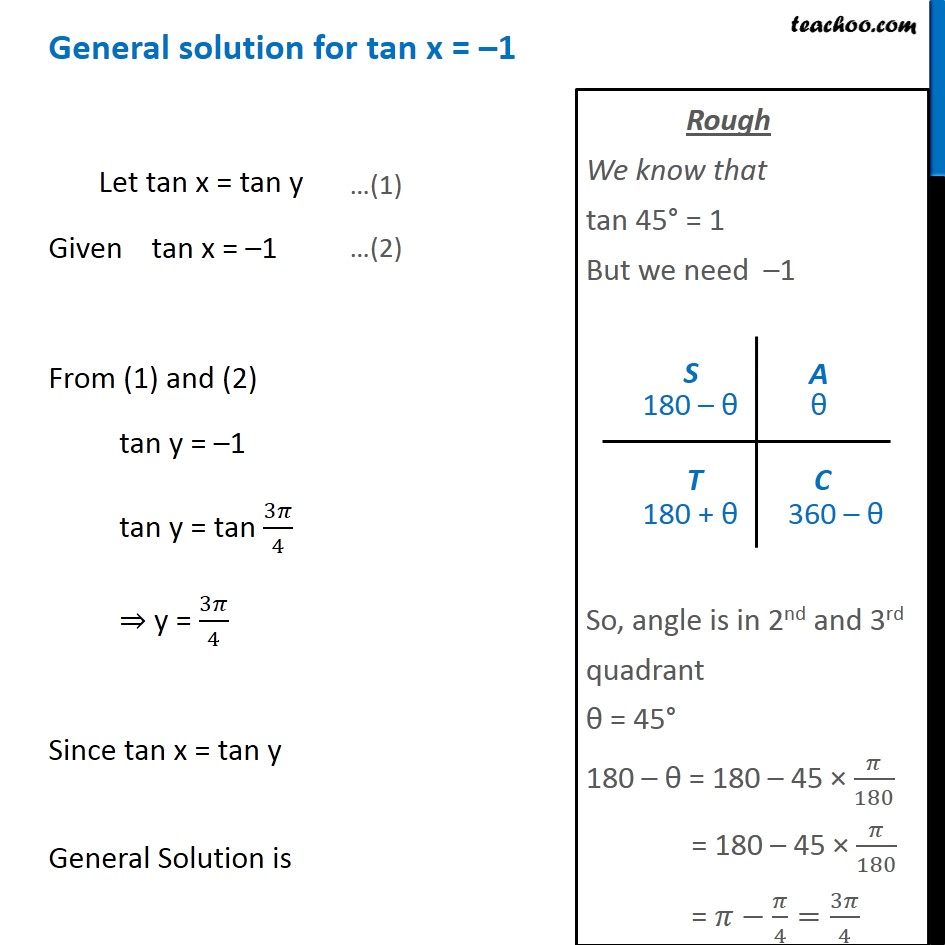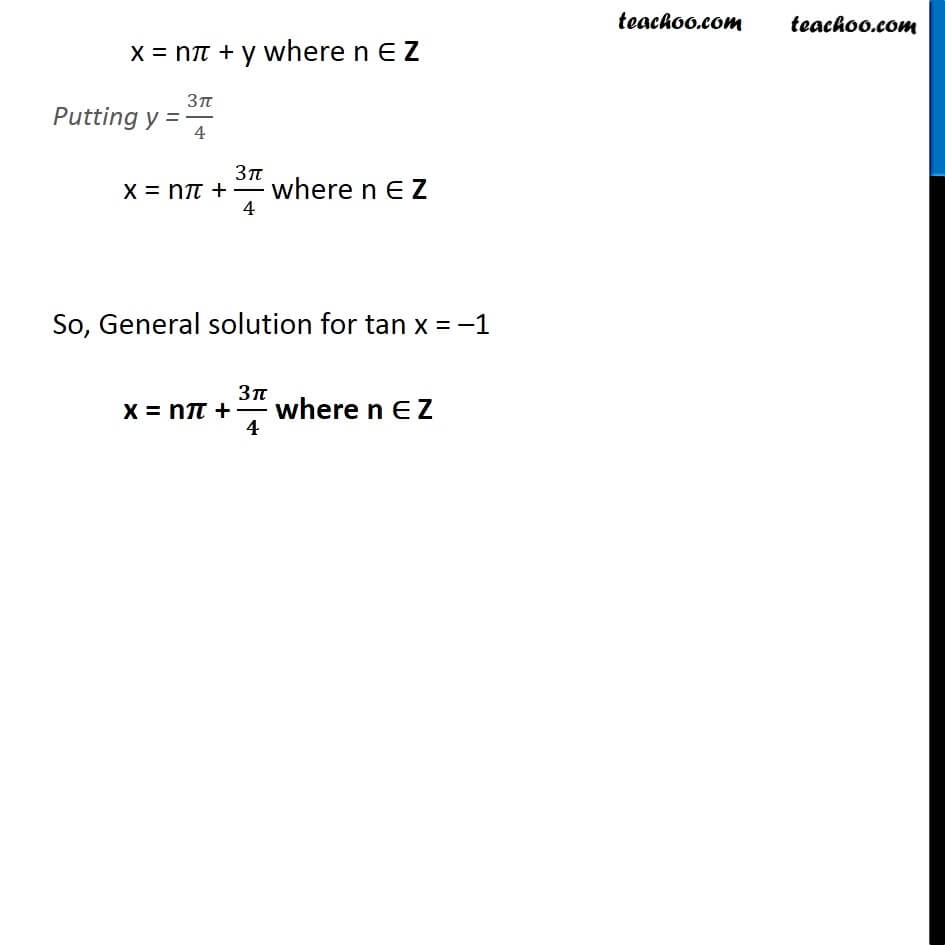Learn in your speed, with individual attention - Teachoo Maths 1-on-1 Class

### Transcript

For general solutions We must learn For sin x = sin y, x = nπ + (–1)n y, where n ∈ Z For cos x = cos y, x = 2nπ ± y, where n ∈ Z For tan x = tan y, x = nπ + y, where n ∈ Z Note: Here n ∈ Z means n is an integer